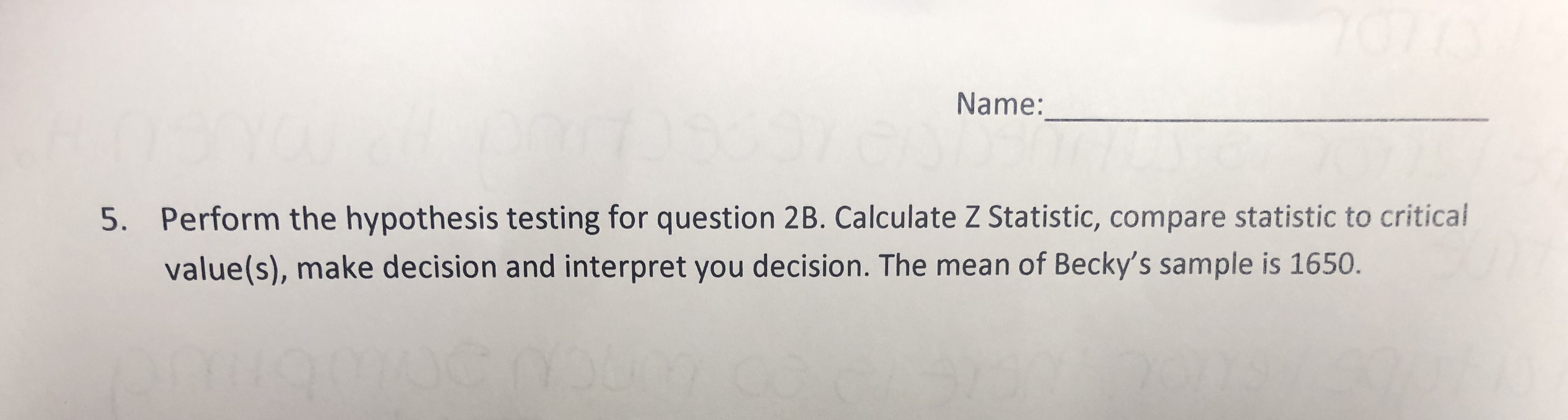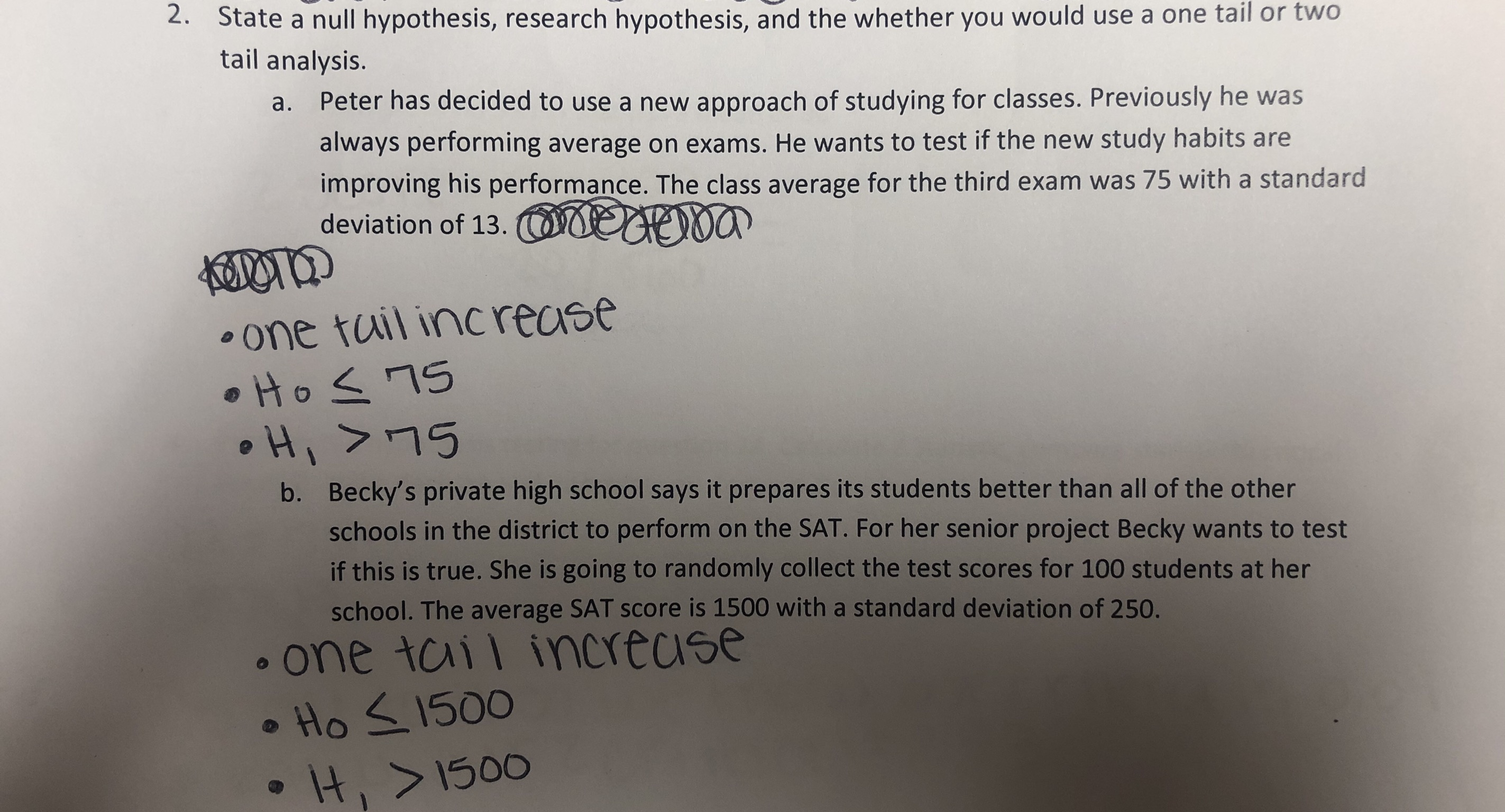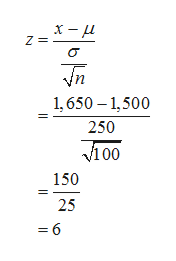Name:Perform the hypothesis testing for question 2B. Calculate Z Statistic, compare statistic to critical5.value(s), make decision and interpret you decision. The mean of Becky's sample is 1650. State a null hypothesis, research hypothesis, and the whether you would use a one tail or twotail analysis.2.Peter has decided to use a new approach of studying for classes. Previously he wasa.always performing average on exams. He wants to test if the new study habits areimproving his performance. The class average for the third exam was 75 with a standarddeviation of 13.one tuil increaseBecky's private high school says it prepares its students better than all of the otherschools in the district to perform on the SAT. For her senior project Becky wants to testb.if this is true. She is going to randomly collect the test scores for 100 students at herschool. The average SAT sco re is 1500 with a standard deviation of 250.one tail inerecseHo S1500t,>1500

Questionhelp_outlineImage TranscriptioncloseName: Perform the hypothesis testing for question 2B. Calculate Z Statistic, compare statistic to critical 5. value(s), make decision and interpret you decision. The mean of Becky's sample is 1650. fullscreenhelp_outlineImage TranscriptioncloseState a null hypothesis, research hypothesis, and the whether you would use a one tail or two tail analysis. 2. Peter has decided to use a new approach of studying for classes. Previously he was a. always performing average on exams. He wants to test if the new study habits are improving his performance. The class average for the third exam was 75 with a standard deviation of 13. one tuil increase Becky's private high school says it prepares its students better than all of the other schools in the district to perform on the SAT. For her senior project Becky wants to test b. if this is true. She is going to randomly collect the test scores for 100 students at her school. The average SAT sco re is 1500 with a standard deviation of 250. one tail inerecse Ho S1500 t,>1500 fullscreen
Step 1

Hypotheses:

Null hypothesis:

H0: µ ≤ 1,500

Alternative hypothesis:

H1: µ > 1,500

Since the alternative hypothesis states µ > 1,500, this test is a right-tailed test.

Critical value:

Consider the level of significance as 0.05.The critical value for 0.05 significance level is 1.96.

Step 2

Calculation:

It is given that X has a normal distribution and σ = 250.

Since it is assumed that X has a normal distribution with known σ, the standard normal is the appropriate sampling distribution.

Test statistic for z-test:

Here, the sample mean, x-bar is 1,650.

Population mean, µ is 1,500.

Population standard deviation, σ = 250.

Sample size, n is 100.

The test statistic for z-test is calculated as 6 from the calculations given below.help_outlineImage TranscriptioncloseZ n 1,650 1,500 250 v100 150 25 =6 fullscreen
Step 3

Computation of P-value:

The P-value for the z-test can be obtained using the excel formula,=NORM.S.DIST(6,TRUE)” and is 1.00.

Th...

Want to see the full answer?

See Solution

Want to see this answer and more?

Our solutions are written by experts, many with advanced degrees, and available 24/7

See Solution
Tagged in

Statistics